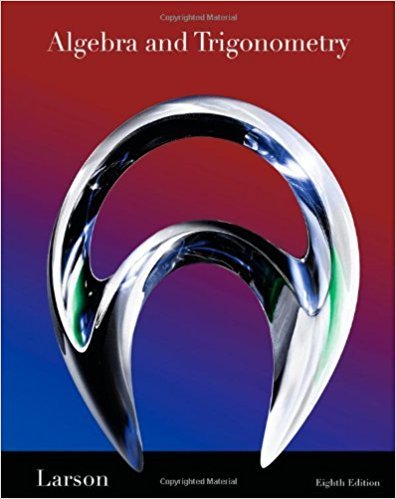×
×

# Solutions for Chapter 10.5: Applications of Matrices and Determinants## Full solutions for Algebra and Trigonometry | 8th Edition

ISBN: 9781439048474Solutions for Chapter 10.5: Applications of Matrices and Determinants

Solutions for Chapter 10.5
4 5 0 362 Reviews
17
1
##### ISBN: 9781439048474

Algebra and Trigonometry was written by and is associated to the ISBN: 9781439048474. Since 77 problems in chapter 10.5: Applications of Matrices and Determinants have been answered, more than 47623 students have viewed full step-by-step solutions from this chapter. Chapter 10.5: Applications of Matrices and Determinants includes 77 full step-by-step solutions. This expansive textbook survival guide covers the following chapters and their solutions. This textbook survival guide was created for the textbook: Algebra and Trigonometry, edition: 8.

Key Math Terms and definitions covered in this textbook
• Cayley-Hamilton Theorem.

peA) = det(A - AI) has peA) = zero matrix.

• Cofactor Cij.

Remove row i and column j; multiply the determinant by (-I)i + j •

• Column space C (A) =

space of all combinations of the columns of A.

• Complete solution x = x p + Xn to Ax = b.

(Particular x p) + (x n in nullspace).

• Iterative method.

A sequence of steps intended to approach the desired solution.

• Left nullspace N (AT).

Nullspace of AT = "left nullspace" of A because y T A = OT.

• Linear combination cv + d w or L C jV j.

• Nilpotent matrix N.

Some power of N is the zero matrix, N k = o. The only eigenvalue is A = 0 (repeated n times). Examples: triangular matrices with zero diagonal.

• Nullspace matrix N.

The columns of N are the n - r special solutions to As = O.

• Particular solution x p.

Any solution to Ax = b; often x p has free variables = o.

• Positive definite matrix A.

Symmetric matrix with positive eigenvalues and positive pivots. Definition: x T Ax > 0 unless x = O. Then A = LDLT with diag(D» O.

• Projection matrix P onto subspace S.

Projection p = P b is the closest point to b in S, error e = b - Pb is perpendicularto S. p 2 = P = pT, eigenvalues are 1 or 0, eigenvectors are in S or S...L. If columns of A = basis for S then P = A (AT A) -1 AT.

• Rank one matrix A = uvT f=. O.

Column and row spaces = lines cu and cv.

• Reflection matrix (Householder) Q = I -2uuT.

Unit vector u is reflected to Qu = -u. All x intheplanemirroruTx = o have Qx = x. Notice QT = Q-1 = Q.

• Right inverse A+.

If A has full row rank m, then A+ = AT(AAT)-l has AA+ = 1m.

• Row space C (AT) = all combinations of rows of A.

Column vectors by convention.

• Schwarz inequality

Iv·wl < IIvll IIwll.Then IvTAwl2 < (vT Av)(wT Aw) for pos def A.

• Semidefinite matrix A.

(Positive) semidefinite: all x T Ax > 0, all A > 0; A = any RT R.

• Triangle inequality II u + v II < II u II + II v II.

For matrix norms II A + B II < II A II + II B II·

• Unitary matrix UH = U T = U-I.

Orthonormal columns (complex analog of Q).

×